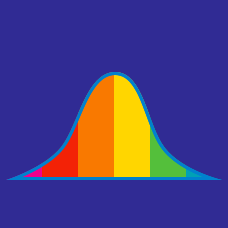Probability

# Understanding Data

#### Challenge Quizzes

A set of an odd number of positive data points has a median of 10 and an inter-quartile range of 5. If all the data points are squared, what is their new median?

You have two sets of corresponding measurements $X = x_1, x_2, \ldots, x_n$ and $Y = y_1, y_2, \ldots, y_n$. Their covariance, $\text{Cov}(X,Y)=12.$ What is the covariance if you double all measurements, i.e. what is $\text{Cov}(2X,2Y)$?

You have a data set with standard deviation 10. What is the minimum value of the standard deviation of the data set obtained by taking the absolute value of all the elements of the original data set?

A set of an odd number of data points has median 5. If we square all the values, what is the new median?

Suppose you have data points $x_1, \ldots, x_n$ with mean 5 and variance 2. If I first multiply them all by three and then square all the new values I get, what is the mean of the data points I end up with?

×